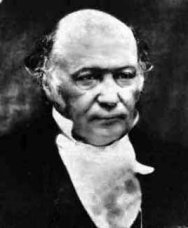# William Rowan HamiltonIf you look through a piece of crystalline calcite, you will see a double image:This effect was discovered by Christiann Huygens in 1690. It is due to the fact that the calcite index of refraction is not isotropic, but depends on direction. So, a light beam incident upon a calcite crystal can be split into two beams which will emerge from the crystal in two different directions, and that results in the familiar double image.

In 1832, the great Irish physicist Hamiltonian made an amazing prediction of a previously unknown optical phenomenon. He predicted that under special circumstances, instead of two beams, an infinite number of beams could be made to emerge from the crystal (in this particular case, not a calcite crystal, but aragonite), and the beams would form a hollow cone. This conical refraction that Hamilton predicted was in fact observed in 1833 by Lloyd.

Hamilton made monumental contributions to optics ( Theory of Systems of Rays ) and mechanics ( On a General Method in Dynamics ), but he considered his greatest work to be his discovery of the mathematical objects known as quaternions .

Hamilton's discovery was made while he was attempting to generalize the complex numbers from two dimensions to three dimensions. The complex numbers are normally represented by x+ iy, with i being a new quantity obeying i*i = -1, and x,y real numbers. He was seeking a generalization to three dimensions by adding, reasonably enough, a single new entity to the complex numbers. So, in effect, this would be akin to a creating new quantity of the form a+ix +jy, with x,y,and a real, and by analogy with the complex numbers, requiring the new entity j to obey j*j = -1. Unfortunately, as was proven later, it is not possible with three terms to do so in a way that retained all the required algebraic properties.

Hamilton achieved the required generalization when he realized he needed to add not one, but two new entities, thus creating a new object of the form a + ix +jy + kz (with j*j = -1 and k*k = -1). This new object could be made to have the correct algebraic properties, and he called it a quaternion. Quaternions have the property of having a non-commutative multiplication, and so from a purely mathematical view are interesting as an example of a skew-field.

In particular, the quaternion multiplication of two unit quaternions, each representing a rotation, can be interpreted as yielding a new quaternion that corresponds to the composition of the two rotations.

Quaternions also have the mathematical advantage of being well defined for all angles. By contrast, in the usual Euler angles description of a rigid body, if one examines the Jacobian of the transformation of angular velocities from the fixed frame to the body frame, one finds it is singular at theta= Pi...and at this angle,  a gyroscope that has been built using Euler angles will exhibit the annoying behavior of gimbal lock. To see an actual example of gimbal lock in such a gyroscope, look at 3:43 in this video.

In computer graphics animation, quaternion interpolation provides an effective means of spherical linear interpolation, or SLERP, as it is known. That is, if you have two points on the unit sphere, SLERP allows you to generate a sequence of points, also on the sphere, (this set of points are also known as "keyframes" in computer animation) beginning with one of the given points and ending on the other point, and also such that the transversing the sequence will be done with a constant velocity.